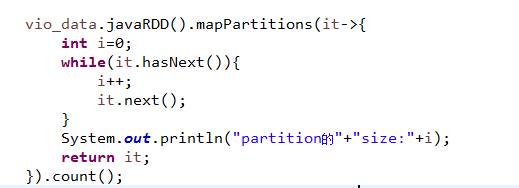• public static int lowerBound(int[] nums, int target) { int left = 0, right = nums.length - 1; while (left <= right) { int mid = (left + right) / 2; if (nums[mid] < target) { left = mid + 1;...
public static int lowerBound(int[] nums, int target) {
int left = 0, right = nums.length - 1;
while (left <= right) {
int mid = (left + right) / 2;
if (nums[mid] < target) {
left = mid + 1;
} else {
right = mid - 1;
}
}
return left;
}

public static int upperBound(int[] nums, int target) {
int left = 0, right = nums.length - 1;
while (left <= right) {
int mid = (left + right) / 2;
if (nums[mid] <= target) {
left = mid + 1;
} else {
right = mid - 1;
}
}
return left;
}

最后一个小于等于目标值
public static int lastLE(int[] nums, int target) {
int left = 0, right = nums.length - 1;
while (left <= right) {
int mid = (right - left) / 2 + left;
if (nums[mid] <= target) {
left = mid + 1;
} else {
right = mid - 1;
}
}
return right;
}

展开全文算法
• 如何加快数据的读取过程 利用SparkSQL读取数据库数据的时候，如果数据量很大，那么...例如下面这样，指定了partitionColumn，lowerBoundupperBound，numPartitions等读取数据的参数。 关于这四个参数的意思，Spark
如何加快数据的读取过程  利用SparkSQL读取数据库数据的时候，如果数据量很大，那么在读取数据的时候就会花费大量的时间，因此，怎么让数据并行读取加快读取数据的速度呢？
在SparkSQL中，读取数据的时候可以分块读取。例如下面这样，指定了partitionColumn，lowerBound，upperBound，numPartitions等读取数据的参数。
关于这四个参数的意思，SparkSQL官方解释是：
Property NameMeaningpartitionColumn, lowerBound, upperBoundThese options must all be specified if any of them is specified. In addition, numPartitions must be specified. They describe how to partition the table when reading in parallel from multiple workers. partitionColumn must be a numeric column from the table in question. Notice that lowerBound and upperBound are just used to decide the partition stride, not for filtering the rows in table. So all rows in the table will be partitioned and returned. This option applies only to reading.numPartitionsThe maximum number of partitions that can be used for parallelism in table reading and writing. This also determines the maximum number of concurrent JDBC connections. If the number of partitions to write exceeds this limit, we decrease it to this limit by calling coalesce(numPartitions) before writing.
public static Dataset<Row> sparkLoad(SparkSession spark, String url, String fullTable,
String partitionColumn, long lowerBound, long upperBound, int numPartitions) {
.option("dbtable", fullTable).option("user", "postgres")
.option("driver","org.postgresql.Driver")
if(partitionColumn != null){
.option("lowerBound", lowerBound)
.option("upperBound", upperBound)
.option("numPartitions", numPartitions);
}
}
从上面的解释来看，分区列得是数字类型；所谓的并行读取其实就是开了多个数据库连接，分块读取的。另外需要注意的是：

Notice that lowerBound and upperBound are just used to decide the partition stride, not for filtering the rows in table. So all rows in the table will be partitioned and returned.

也就是说，这些参数的设置不会过滤数据，所以sql中读取了多少数据，那么返回的就是多少条数据，lowerBound和upperBound并不会过滤数据。那么如果说设置的lowerBound偏大（可能读取的数据中分区列的值比这个小），或者设置的upperBound数值设置的大小偏小（可能读取的数据中分区列中最大的值比upperBound大），这个时候数据是怎么读取和返回的呢？
举个例子：
如果一个表分10个分区读取，id为分区列，其值从0-101，但是设置的lowerBound是1，upperBound是100，那么读取的规则如下：  第一个分区：select * from tablename where id<=10;  第二个分区：select * from tablename where id >=10 and id<20;  第三个分区：select * from tablename where id >=20 and id <30;  ……  第十个分区：select * from tablename where id >=90；
这样查询不会遗漏结果，但是至于上面的边界条件，等于号是在左边还是在右边这个还不太清楚，笔者也没有研究。其实这个如果要研究的话，也很简单，用一个测试表分区后，分别读取每个分区的数据条数并打印输出，就可以知道了。  思路如下：但是笔者觉得没有研究的必要，如果对项目没有任何bug上的影响，就不需要研究这么细节，对技术没有什么帮助。
展开全文SparkSQL
• c++中 lower_bound upper_bound非常经典，我们用java实现一下。 /** * @param arr * @param value * @return 第一个大于等于value的数的坐标 */ int lowerBound(int[] arr, int value) { int l = 0, r =...
c++中 lower_bound 和 upper_bound非常经典，我们用java实现一下。
 /**
* @param arr
* @param value
* @return 第一个大于等于value的数的坐标
*/
int lowerBound(int[] arr, int value) {
int l = 0, r = arr.length - 1;
while (l <= r) {
int m = ( l+r) / 2;
if (arr[m] < value) {
l = m + 1;     // 如果m位置的元素太小，直接把左边界跳到m+1
} else { // 相当于 arr[m] >= value
r = m - 1;  // 虽然m有可能是目标解，直接m-1会错过，但是最后如果在 l 和 m -1 里面找不到， l会取“m+1”，跳出循环，这里的“m+1“其实就是错过的目标解、
}
}
return l;
}

/**
* @param arr
* @param value
* @return 第一个大于value的数的坐标
*/
int upperBound(int[] arr, int value) {
int l = 0, r = arr.length - 1;
while (l <= r) {
int m = ( l+r) / 2;
if (arr[m] <= value) {
l = m + 1;
} else {  // arr[m] > value
r = m - 1;
}
}
return l;
}

展开全文二分查找 java
• 使用二分查找实现lower_...upperBound找到大于当前元素的最小元素下标 1, 3, 4, 5, 6, 6, 7, 8, 8, 9, 10 ^ ^ lowerBound(int[] a, int l, int r, int target) upperBound(int[] a, int l, int r, int target) lo
使用二分查找实现lower_bound()和upper_bound()操作
lowerBound找到 >= target的第一个元素下标upperBound找到 > target的第一个元素下标
lowerBound
// 找到第一个大于等于target的元素的下标
// 如果target大于最大的数字，返回最大的数字
public int my_lower_bound(int l, int r, int target, int[] a){
if(target > a[r-1]) return r;
while(l < r){
int mid = l + ((r - l) >> 1);
if(target > a[mid]){
l = mid + 1;
}else{
r = mid;
}
}
return l;
}

upperBound

// 找到第一个大于target的元素的下标
// 如果target大于最大的数字，返回最大的数字
public int my_upper_bound(int l, int r, int target, int[] a){
if(target > a[r-1]) return r;
while(l < r){
int mid = l + ((r - l) >> 1);
if(target >= a[mid]){
l = mid + 1;
}else{
r = mid;
}
}
return l;
}

使用

m

i

d

=

l

+

(

(

r

−

l

)

>

>

1

)

mid = l + ((r-l) >> 1)

是为了防止溢出
展开全文数据结构 java
• 关键概念 partitionColumn：分区字段，需要是数值类的（partitionColumn must be a numeric column from the table in question.），经测试，除整型外，... upperBound：上界，必须为整数 numPartitions：最大分...spark
• lowerbound和upperbound可以直接使用，无需特殊判断
• lower_bound算法要求在已经按照非递减顺序排序的数组中找到第一个大于等于给定值key的那个数，其基本实现原理是二分查找，如下所示： 其中 r = nums.length public static int lowerBound(int []nums,int l,int r...
• set中的元素是有序的 set中find不到元素时返回set.end()。否则返回他的迭代器 lowerbound返回大于等于他的...upperbound返回大于他的第一个元素 。时间复杂度基本为o（n）由此可以实现有序插入寻找两边最近的元素stl-set
• up = upper_bound(v1.begin(), v1.end(), 3); cout *up ; vector<int> v2 = {0, 1, 5, 3, 2, 1, 4, 7, 6}; vector<int>::iterator lo; lo = lower_bound(v2.begin(), v2.end(), 3); cout *lo ; ...c++ c++ c++
• 二分查找算法可以解决最简单的二分查找问题：a数组单调递增，并且其中...为了解决这些问题，C++ STL提供了两个特别好用的函数：lower_bound()uppper_bound()。假设a是一个数组，n是数组长度，lower_bound(a, a+n...
• 例如下面这样，指定了partitionColumn，lowerBoundupperBound，numPartitions等读取数据的参数。简单来说，就是并行读取。 关于这四个参数的意思，SparkSQL官方解释是： Property Name Meaning ...
• 转载至某个比较好看的博客：关于lower_bound( )和upper_bound( )的常见用法 下面的内容： lower_bound( )和upper_bound( )都是利用二分查找的方法在一个排好序的数组中进行查找的。 在从小到大的排序数组中...
• lower_bound( )和upper_bound( )都是利用二分查找的方法在一个排好序的数组中进行查找的。在从小到大的排序数组中，lower_bound( begin,end,num)：从数组的begin位置到end-1位置二分查找第一个大于或等于num的数字，...
• lower_bound( )和upper_bound( )都是利用二分查找的方法在一个排好序的数组中进行查找的。 在从小到大的排序数组中， lower_bound( begin,end,num)：从数组的begin位置到end-1位置二分查找第一个大于或等于num的...
• 1. 问题引出 ...// http://www.cplusplus.com/reference/algorithm/upper_bound/ // 从mvOrderedWeights找出第一个大于w的那个迭代器 // 这里应该使用lower_bound，因为lower_bound是返回小于...
• //4.以某一特定位置来截取字符串var string = " https://www.baidu.com"let range = string.range(of: "baidu")let lowerStr = string.substring(to....lowerBound)!)//结果为： https://www.print("to lower string...
• 由于对于二分法一直都不是很熟悉，这里就用C++中的lower_bound和upper_bound练练手。这里用python实现 lower_bound和upper_bound本质上用的就是二分法，lower_bound查找有序数组的第一个小于等于目标数的，upper_...
• lowerBoundupperBound /** *用while代替递归的二分查找 * @param array * @parram target * @return target的位置 */ public static int binarySearch(int[] array,int target){ int low = 0; int high =...二分查找 算法 java leetcode
• 1：lower_bound()和upper_bound()都是利用二分查找的方法实现的. 1：lower_bound(begin,end,val)；该函数可以在一个已经排序好的序列中，从begin位置一直到end位置前一个来查找第一个大于等于val值的位置，返回这个...数据结构
• 以前用这两个函数的时候，简单看了几句别人的博客，记住了大概，用的时候每用一次就弄混一次，相当难受，今天对照着这两个函数的源码自己的尝试发现：其实这两个函数只能用于 “升序” 序列。 为什么还要加个引号...c 迭代器 STL
• 介绍结构体数组包含结构体的vector怎么样使用lower_bound进行二分查找，upper_bound同理。 前提： lower_bound:返回数组中第一个大于等于该元素的下标，int aa = lower_bound(array,array+arrayLen,num) - array...vector
• 使用这两个函数都需要包含对应...upper_bound(查找的起始位置，查找的终止为止，需要查找的数 )是返回第一个大于等于需要查找的数的数的地址 注意上面的两种用法都需要原数组是小到大排列的有序数组 可以通过修改比c++
• 前几天复习二分然后百度了下lower_bound()和upper_bound()函数，现在将我的理解说下，先贴出我写的代码！ #include using namespace std; int lower_bound(int *a, int n, int value) //二分查找第一个大于或等于...
•  对于upper_bound来说，返回的是被查序列中第一个大于查找值的指针，也就是返回指向被查值&gt;查找值的最小指针，lower_bound则是返回的是被查序列中第一个大于等于查找值的指针，也就是返回指向被查值&gt;...
• 1.lower_bound(nums, target) 在非递减数组nums中，lower_bound(nums, target)返回第一个大于等于target的值得位置，如果nums中元素均小于target（即不存在>=target的元素），则返回nums的长度（即target如果要......